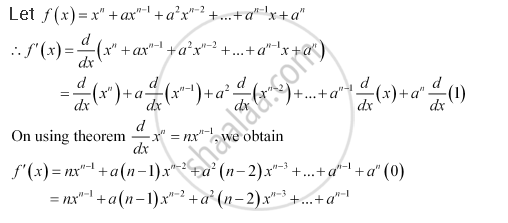Share

# Find the Derivative of X^N + Ax^(N-1) + A^2 X^(N-2) + ...+ A^(N -1) X + A^N For Some Fixed Real Number A - Mathematics

#### Question

Find the derivative of x^n  + ax^(n-1) + a^2 x^(n-2) + ...+ a^(n -1) x + a^n for some fixed real number a

#### SolutionIs there an error in this question or solution?

#### APPEARS IN

NCERT Solution for Mathematics Textbook for Class 11 (2018 (Latest))
Chapter 13: Limits and Derivatives
Q: 6 | Page no. 313

#### Video TutorialsVIEW ALL 

Solution Find the Derivative of X^N + Ax^(N-1) + A^2 X^(N-2) + ...+ A^(N -1) X + A^N For Some Fixed Real Number A Concept: Derivative - Derivative of Polynomials and Trigonometric Functions.
S